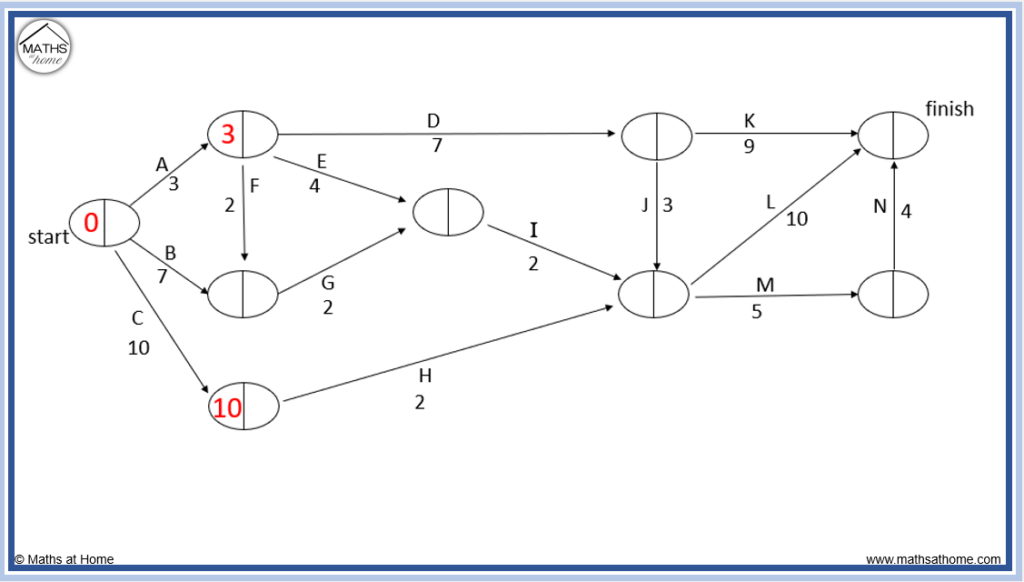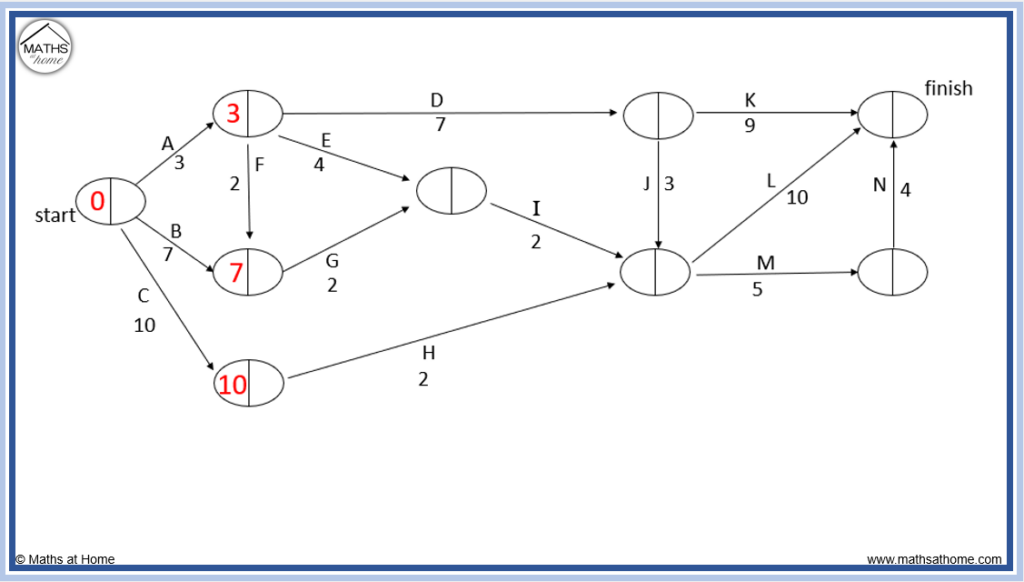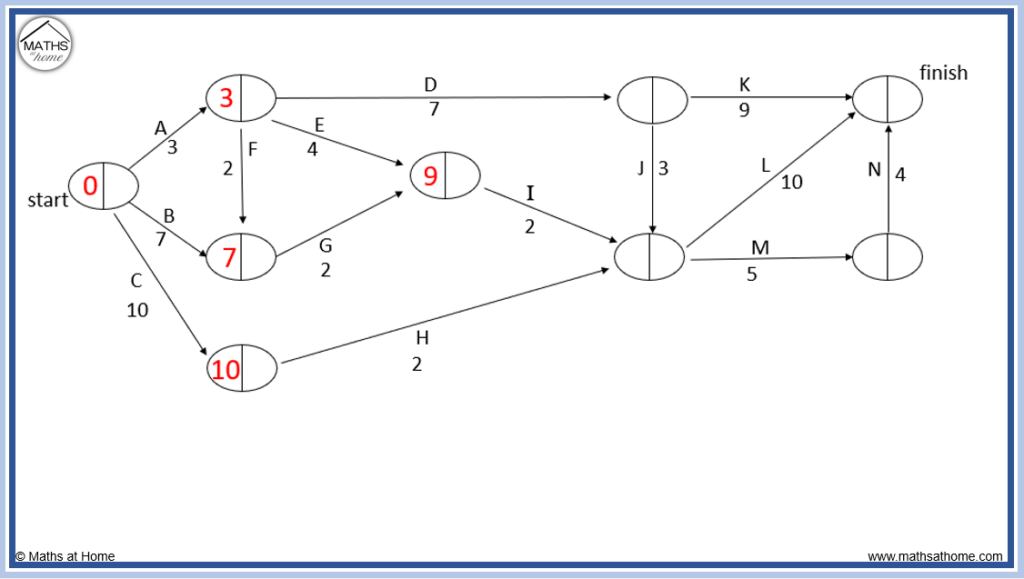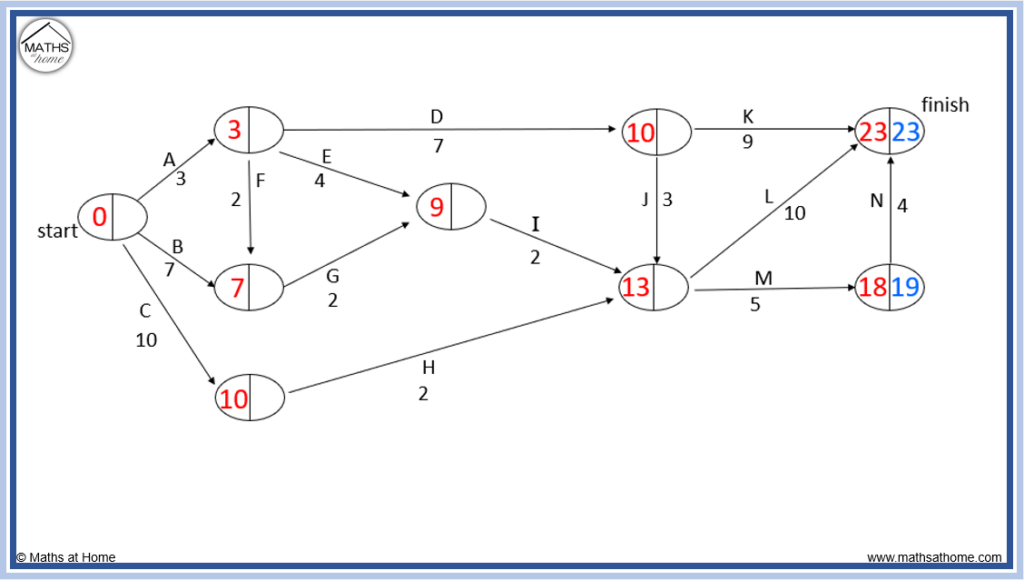# Forward/Backward Scan: How to Find the Critical Path

## What is the Critical Path?

The critical path is the longest sequence of tasks from project start to finish. The sum of the times on the critical path is equal to the project minimum completion time. The critical path contains tasks that have no slack time. That is, these tasks must be completed on time to avoid delaying the project.

Here is an example of a critical path within a network.

The critical path is listed as A, D, J, L.

The sum of the times of tasks A, D, J and L are 3 + 7 + 3 + 10 = 23 minutes. The minimum completion time of the project is 23 minutes. This means that the earliest time in which the project can be completed is 23 minutes.

In each node, two numbers are listed.

In the left of the node, we have the earliest time that this task can be started.

In the right of the node, we have the latest time that this task can be started.

Critical tasks are tasks which must be completed immediately to avoid delaying the project. Critical tasks are tasks that have an earliest start time equal to the latest start time. That is, there is no slack time.

Critical path analysis is used in project management to identify critical tasks. These tasks must be completed without delay in order to avoid delaying the overall project completion time. The critical path method (CPM) can be used to make projects more efficient by identifying tasks that should be completed more quickly. The purpose of this is to reduce the minimum completion time and reduce the likelihood of bottlenecks or delays.

Every project must have a critical path. Sometimes multiple critical paths exist with the same minimum completion time.

If a project has already commenced and is suffering many delays, it is possible for there to be negative slack time or no tasks in which the slack time is zero. In this case, the path with the least slack time should be selected as the critical path. A near-critical path is a path that has a similar completion time to the critical path itself.

## How to do a Forward and Backward Scan

To complete a forward scan, we find the longest path to each node. We write this largest number in the left of the node, which tells us the earliest starting time for the following task. To complete a backward scan, work from the finish node backwards, subtracting each edge. The path that makes the smallest number is used with this number written in the right side of the node.

The forward and backward scanning method is used to find the critical path in a network.

### Forward Scan

To do a forward scan:

1. From the starting node, add on the lengths of the following edges and write the longest path to get to the next nodes.
2. Continue to add on the lengths of the edges from each node, only writing the longest number to get to each new node.
3. The time written in the final node is the minimum completion time.

Here is an example of completing the forward scanFrom the starting node, we can only take the task A to the top node. We write 3 in the left of the node.

We can only take task C to get to the bottom node, so we write 10 in this node.To get to the next node, there is a choice of following task F from the node with a 3 above (3+2=5) or alternatively to take task B (7 minutes).

We write 7 minutes as it is the larger number.To get to the next node, there is a choice of taking task E from the node with a 3 (so 3+4=7) or taking task G from the node with a 7 (so 7+2=9).

We write 9, the longest path.

Following task I, we have 9+2=11 and following task H, we have 10+2=12.

However, the longest route to the next node is taking task J from the node above and we write 10+3=13 inside.

### Backward Scan

To do a backward scan:

1. Start at the finish node and travel against the task directional arrows.
2. Subtract the duration of each task, only writing the smallest number to get to each node.
3. For paths that produce a larger number, this is written on the outside of the node.
4. Continue until a zero time is written in the starting node.

Here is an example of completing the backward scan.From the forward scan, the minimum completion time was found to be 23. This time is written in the right of the final node.

Going backwards against task N, 23-4=19, which is written in the next node.

Following task M, 19-5=14 and this is written on the outside of the node as 14 is larger.

We only write the smaller value inside the node.

10 is writen inside as it is smaller. 14 is written on the outside.

Longer paths of task E (11-4=7) and task F (9-2=7) are written on the outside of this node.

Finally, following task A, 3-3=0. This is written in the start node as the start node always contains (0,0).

Following task B, 9-7=2, which is written on the outside by task B.

Following task C, 11-10=1, which is also written on the outside by task C.

Once the start node contains (0,0), the backward scan is complete.

The critical path is shown by connecting the nodes that have the same numbers in them following the forward and backward scan.

In this example, the critical path is A, D, J, L passing from (0,0) to (3,3) to (10,10) to (13,13) to (23,23).

## How to Calculate Slack Time for a Task

Slack time (or float time) is the amount of time that an individual task can be delayed without delaying the overall project completion time. To calculate the slack time, subtract the earliest start time from the latest start time.

The times for each task are found in the node before the task. The earliest start time is written in the left side of this node and the latest start time is written in the right of this node.

Slack time = latest start time – earliest start time.

For example, if a task has an earliest start time (EST) of 3 minutes and a latest start time (LST) of 5 minutes, the slack time is 5 – 3 = 2 minutes.

A slack time of 2 minutes means that this task can be delayed by up to a maximum of 2 minutes without delaying the overall project completion time.

A delay of 1 or 2 minutes will not delay the project completion time, however a delay to this task beyond this will delay the minimum completion time by the extra amount beyond 2 minutes.

Delay to Minimum Completion Time = Task Delay – Task Slack Time

A delay to the task of 3 minutes will delay the project minimum completion time by 3 – 2 = 1 minute.

A delay to the task of 10 minutes will delay the project minimum completion time by 10 – 2 = 9 minutes.

If a number is written outside of the node, use this as the latest start time instead of the latest start time inside the node when calculating slack time.

In the example below, the slack time is calculated by 7 – 3 = 4 minutes rather than 5 – 3 = 2 minutes.

Below is an example of how to find the slack time and delays to a project on a network diagram.

### Slack Time Example

The network below shows the earliest and latest start times for each task.

We will look at some different nodes to calculate their slack times.

Considering the slack time for task G, we look at the node before it containing (7,9). The earliest start time is 7 and the latest start time is 9.

9-7=2 and so, the slack time for task G is 2 minutes.

We can delay task G by 2 minutes with no knock-on effects but not by 10 minutes.

This means that if task G is delayed by 10 minutes, then 10-2=8 minutes will be added on as a delay.

The minimum completion time increases by 8 minutes. The new completion time is 23+8=31 minutes.

Considering task E, the node before it contains (3,3) with a 7 on the outside.

In this case, we take the left number of 3 as the earliest start time but the 7 on the outside is taken as the latest start time instead of the rightmost 3 inside the node.

The slack time is therefore 7-3=4 minutes.

This means that if E is delayed by 2 minutes, there are no delays to the project as we can delay task E by up to 4 minutes with no knock-on effects.

The minimum completion time would remain as 23 minutes.

## How to Find the Critical Path

The critical path is the longest path through a network from start to finish. Tasks on the critical path have an earliest start time equal to their latest start time. That is, they have zero slack time.

For example, consider the network below in which the forward and backward scan has taken place.

The critical path can be found by connecting the nodes in which the earliest start time is equal to the latest start time.

That is, where the numbers in the nodes are the same.

The critical path is A – D – J – L and is shown on the network below.

If the earliest start times (EST) and latest start times (LST) are provided in the prerequisite table, then the critical path can be seen without needing to draw the network or perform a forward scan.

Critical tasks are found where the EST = LST.

In this prerequisite table, the critical tasks are A, D and F.

Therefore, the critical path is A-D-F.

Only a forward scan is necessary when finding the critical path. The critical path is simply the longest path from start to finish.

The critical path is found by calculating the longest path through the network.

In this example we have A – F – G – I – M – N.

The total time is 6 + 2 + 4 + 5 + 5 + 4 = 26 minutes.

## Critical Path with Dummy Activity

Dummy activities (dummy links) have zero duration. Complete the forward scan process as usual to find the critical path but add zero when travelling down a dummy link. If the path taken by the dummy link is larger than the alternate paths, this number must be written as the earliest start time for the next node.

An example of the forward scan is shown in the network below containing a dummy link.

Taking the path of task B is longer than going via task A and then the dummy link. Therefore the 5 from task B is written in the node and the dummy link did not affect the forward scan or the critical path.

In the following example, the dummy link is used as part of the forward scan.

Following task A and then task B we arrive at a node with 7 minutes. Then following the dummy link, the 7 is carried to the next node as its earliest start time.

This is because 7 is the longest path to this node, larger than taking A, C and E, which is 1 + 1 + 2 = 4.

The critical path for the above network does not involve the dummy link.

The critical path is A – D – G – J – M with a total time of 1 + 6 + 4 + 5 + 3 = 19 minutes.

## Changing the Critical Path

If a task on the critical path is made shorter in duration, another critical path may arise instead and the critical path will change. The minimum completion time will be reduced. Speeding up a task that is not on the critical path will not change the critical path or reduce the completion time of the project.

Considering the original network below. The critical path is A – D – G – J – M.

If a project manager considered this network, they may allocate more staff or resources to a task on the critical path in order to speed it up and reduce the overall completion time.

Speeding up a task that is not on the critical path will not reduce the minimum completion time of the project.

For example, if task D is reduced from 6 minutes to 1 minute, the critical path will change to B – F – L as shown in the network below.

If a task on the critical path is reduced in time, a new forward and backward scan should be completed to identify the new critical path.

We can see that the new minimum completion time is now 15 minutes. The previous time before the change was 19 minutes.

Despite reducing the task D by 5 minutes, only 4 minutes were saved in the overall minimum completion time. We must perform the forward and backward pass method to find the total reduction in task time.

When deciding which task to speed up, tasks on the critical path should be chosen. To reduce the minimum completion time the most, the longest tasks on the critical path should be reduced in duration.

In general, a forward and backward scan should be completed to decide if a task lies on the critical path.

Some tasks may always lie on the critical path. That is if there are no parallel tasks that can be done simultaneously. If a task lies on every path from start to finish, this task must lie on the critical path.

For example, task L below must lie on the critical path because all paths from start to finish must pass through task L. There are no alternative routes.

## Assumptions of the Critical Path Method

The following assumptions are made in the critical path method (CPM)

• All tasks must be completed
• All tasks always take the stated durations
• Tasks can be completed simultaneously
• A task cannot be started until its prerequisite tasks are complete